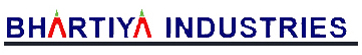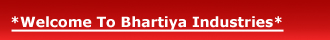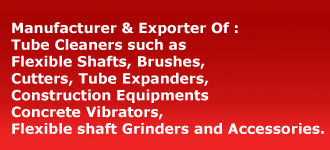Our Product Range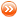# Hand Torque Multiplier

Bhartiya Torque Multipliers are Heavy Duty Tools used to increase the Torque produced by Torque Wrenches. They have been developed to meet the high Torque requirements and keeping in view space restrictions in Torque Wrench applications. Torque multipliers are suggested for either tightening of high tensile bolts or loosening Rusty & Frozen but bolts.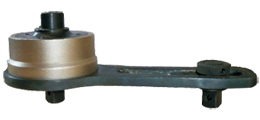FEATURES: These are precision Gear units designed for multiplication of input Torque multipliers are recommended to be used where very high Torque (over 100 kg mtr.) Are required to minimize input effort. As such the use of Torque multipliers reduces the manpower required as well as the operators fatigue.
The multiplier has a gear mechanism by which the Torque applied on the input side is multiplied in a particular ratio. Bharatiya Torque Multipliers are available in Multiplication Factors of 5:1 & 25:1. Torque Multiplier covers Torque requirement from 100 kg mtr. To 925 kg mtr. (750 lb. Ft to 7000 lb.ft)

The multiplier has a gear mechanism by which the Torque applied on the input side is multiplied in a particular ratio. Bharatiya Torque Multipliers are available in Multiplication Factors of 5:1 & 25:1. Torque Multiplier covers Torque requirement from 100 kg mtr. To 925 kg mtr. (750 lb. Ft to 7000 lb.ft)APPLICATIONS: Bhartiya Tool Torque Wrenches are recommended to be used at the Input end to achieve an exactly calculated output Torque so the fluctuation of efficiency remains extremely small over period. It is extensively used in large Petrochemicals and Refineries.

Designed By: MIPL | Hosted by : MID |  Promoted by : GID